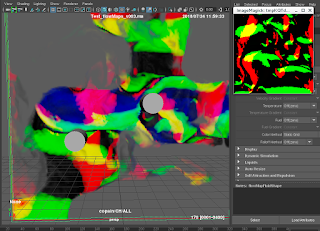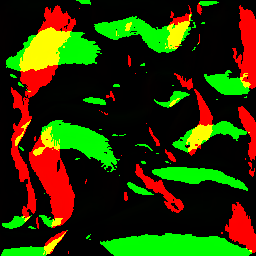### Generating Flow Maps with Python and Maya Fluids

Often I come across a problem, and while not knowing how the solution will be used, I'm certain it will be used in the future.

So. Generating flow maps for Unity.  A flow map is a colored image which stores vector (motion) information.   It's commonly used to guide the flow of water effects using bump or displacement maps. Here's an example below.

The RG image at the bottom represents the direction and the magnitude of the flow.

Since I like using Maya fluids, I wanted to create a flow map unsing a 2d fluid container. The concept: Get the XY velocity of a fluid, and pass that to the RG color channels of an image.  Here's a simple fluid simulation with collisions and a little python script I wrote to recolor the fluid:

import maya.cmds as cmd
def colorflud(multi = 1, res = 256, den = 0.5):
fluidShape = 'flowMapFluidShape'
fludArr = cmd.getAttr('%s.fieldData.fieldDataVelocity' % fluidShape)
massArr = cmd.getAttr('%s.fieldData.fieldDataMass ' % fluidShape)
i = 0
for y in (range(res)):
for x in (range(res)):
cmd.setFluidAttr(fluidShape, at='color', vv = [(fludArr[i] * multi), (fludArr[i]* multi), (massArr[i]*den)], xi=x, yi=y, zi=0 )
i+=1

So you can see that we get a nice visualization of the fluid velocity vector in the viewport, now of course we can't visualize negative values, which come through as black, but that's ok since we're going to pass that data to an image.  Here's what the test looks like in motion (In this case red and green are motion vectors and blue represents density.)

The next step was to pass this color value directly to an image.  To do that I had to use numpy to create an array, and PIL which is an imaging utility.  My first tests were spitting out garbage images with random colors, but eventually after a lot of copying and pasting from forums I found the formula that worked.  Almost.
``````import maya.cmds as cmd
from PIL import Image
import numpy as np

fluid = 'flowMapFluidShape'
res = cmd.getAttr('%s.baseResolution' % fluid)

fludArr = cmd.getAttr('%s.fieldData.fieldDataVelocity' % fluid)
massArr = cmd.getAttr('%s.fieldData.fieldDataMass ' % fluid)

#create a 2D array of the same size as the fluid

c_array = np.zeros([res, res, 3],dtype = np.uint8)

#fill the array with velocity and mass data
i=0
for y in reversed(range (res)):
for x in range (res):
c_array[y, x] = [fludArr[i], fludArr[i], massArr[i]]
#visualise the fluid in viewport
#cmd.setFluidAttr(fluid, at='color', vv = [(fludArr[i] ), (fludArr[i]), (massArr[i])], xi=x, yi=y, zi=0 )
i+=1

img = Image.fromarray(c_array, 'RGB')
img.save('my.png')
img.show()

help(Image.fromarray)``````Compare the visualized flow map to the output image (below)No gradiation, and values are reversed!
So in a nutshell, the image doesn't look like the fluid!  There's no gradiation, no softness, and from what I can tell, no negative values either!  I can't use this as a flow map.  I tried multiple combinations of data types in the Numpy and Image functions, but no luck.  So here's where I threw the problem up on some CG boards to see if anyone has a clue on how to pass velocity data from a fluid to a texture file properly.  I know this will come in handy for generating animated flow maps in the future - if I can only get it working.  Meanwhile time to watch some Houdini tutorials, apparently this sort of thing is a piece of cake in Houdini.